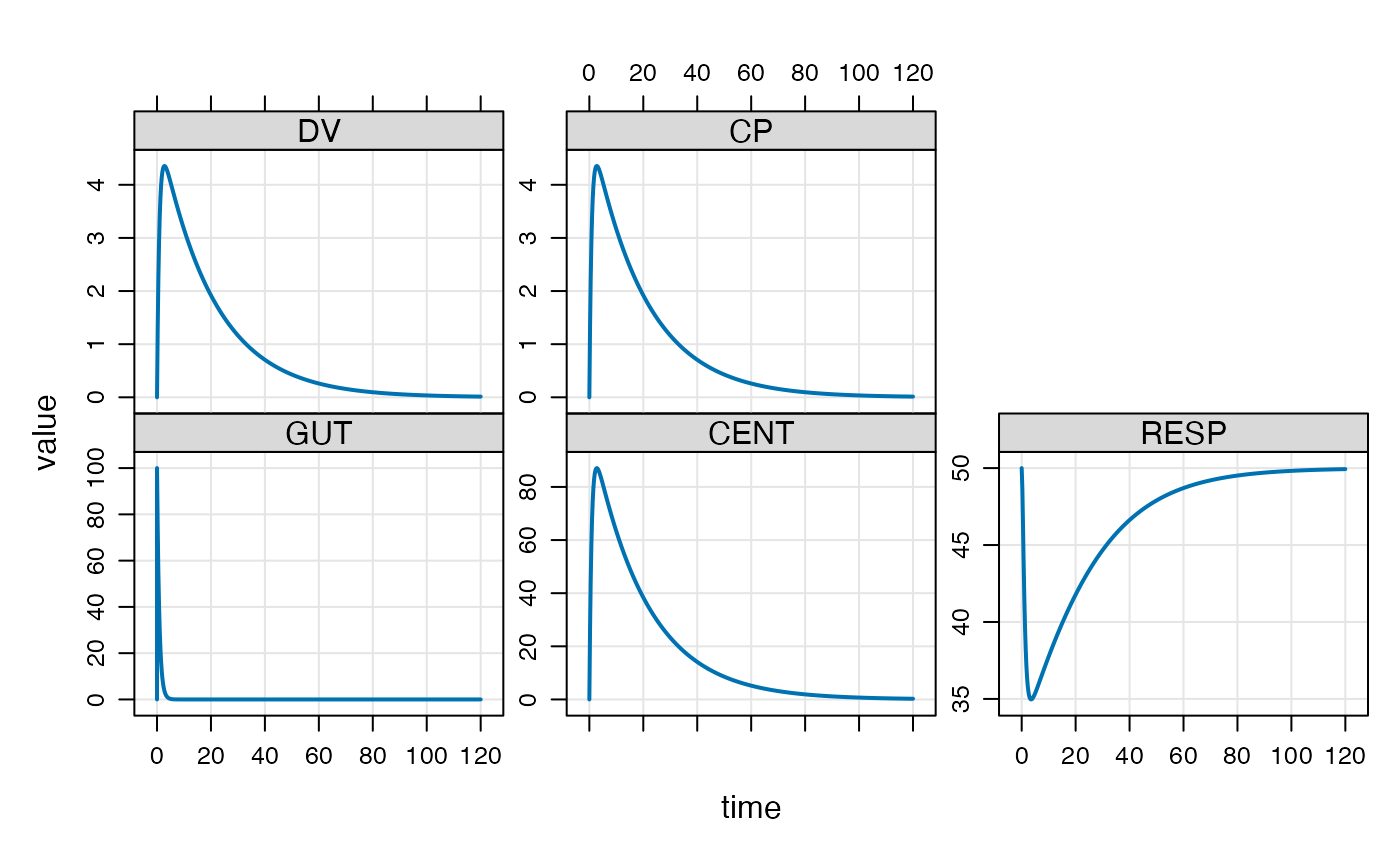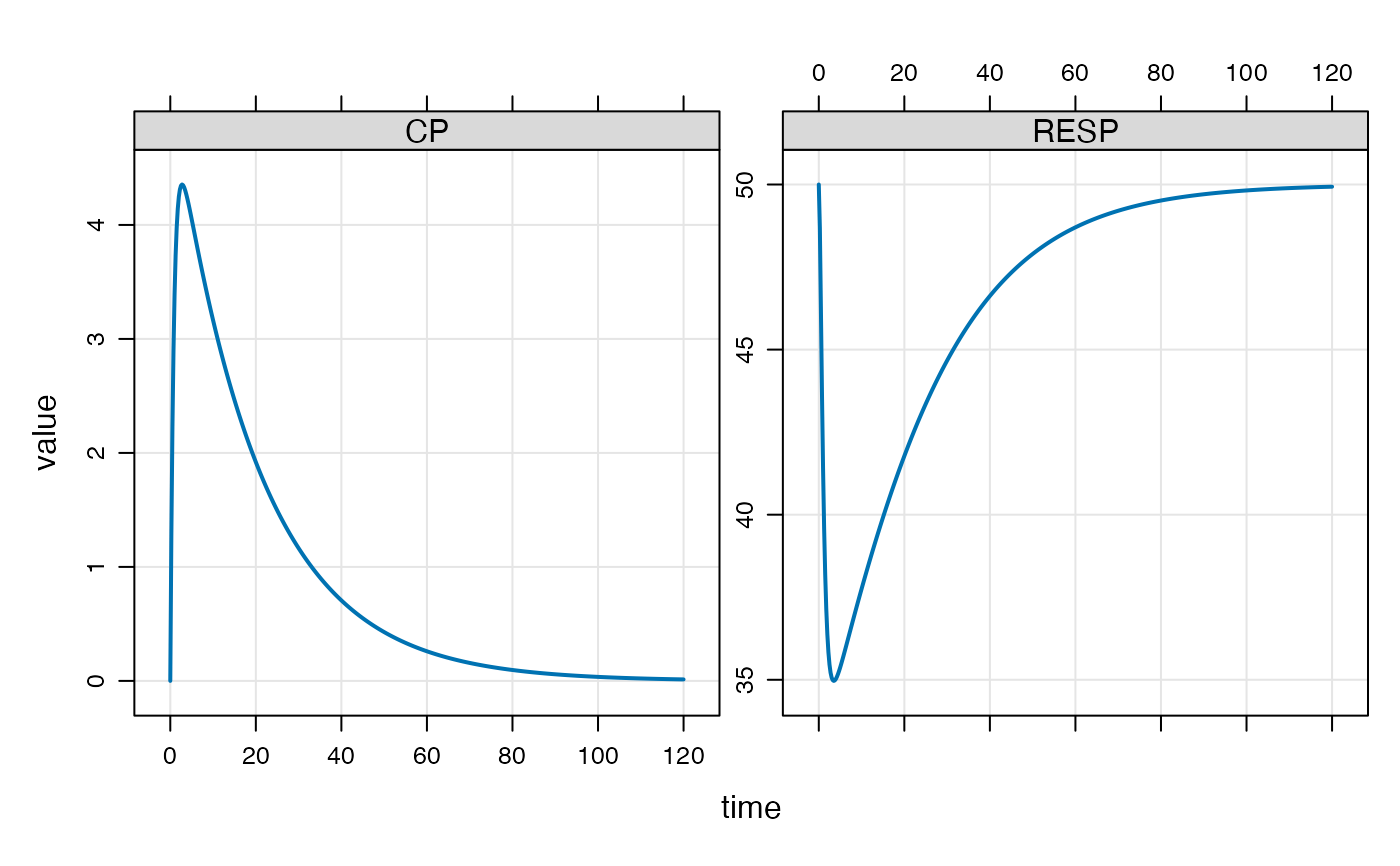Plot data as an mrgsims object

## Usage

plot_sims(.data, ..., .f = NULL, .dots = list())

## Arguments

.data

a data frame

...

unquoted column names to plot on y-axis

.f

a formula to plot

.dots

extra arguments passed to lattice::xyplot

## Details

This function is only intended for use with data frames that were created by modifying an mrgsims object.

## Examples


mod <- mrgsolve::house() %>% ev(amt = 100)

out <- mrgsim(mod)
out_df <- dplyr::mutate(out, time <= 72)

plot(out)plot_sims(out, CP, RESP)if (FALSE) {
plot_sims(out, .f = ~ CP + RESP)
plot_sims(out, .f = CP + RESP ~ time)
}